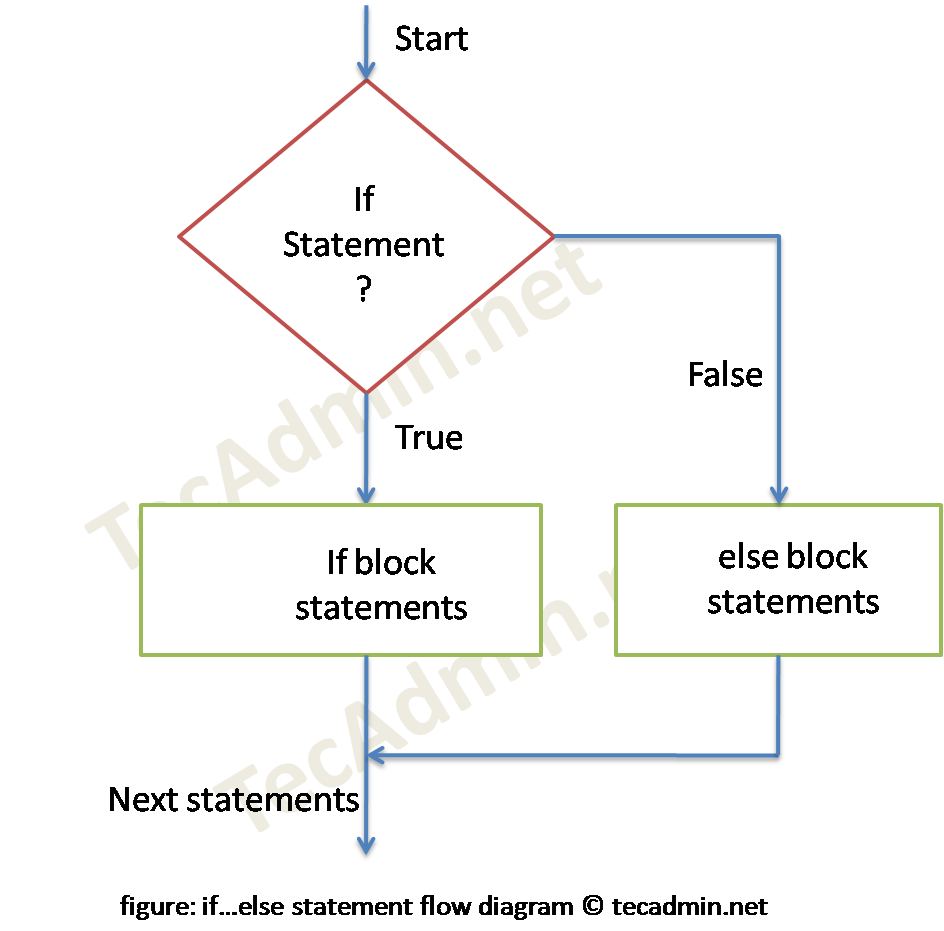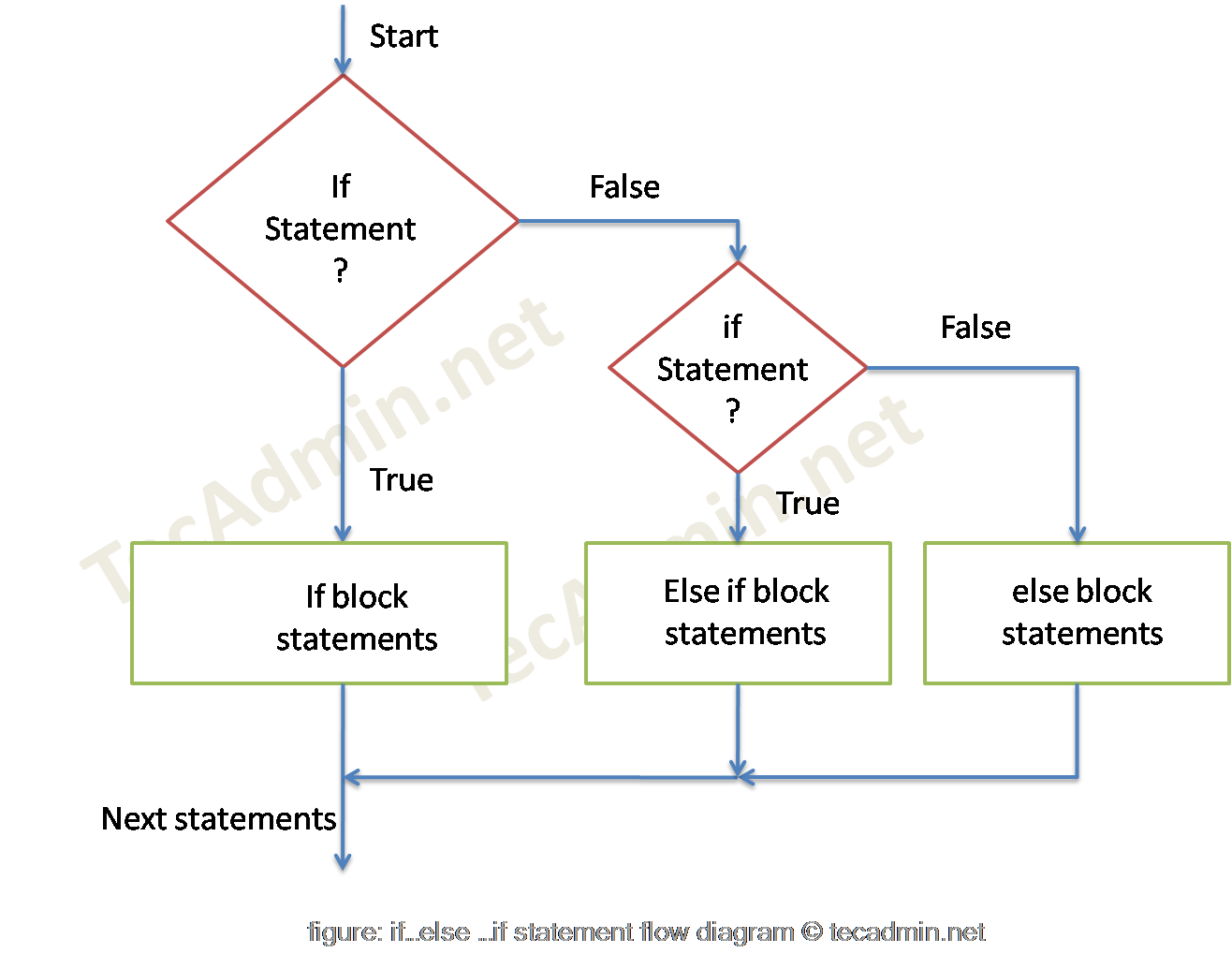1. Home
2. Bash
3. Bash Tutorial
4. Bash – If-else

# Bash if else Statment

If-else is the decision making statements in bash scripting similar to any other programming. Where execution of a block of statement is decided based on the result of if condition. If it evaluates a condition to true, then if block code is executed, on the false condition, the else block code is executed, which is optional.

Syntax:

basically, there are 4 types of if statements.

• if statement
• if-else statement
• nested if statement

## 1. Bash – if Statement Example

This is the basic if condition, where the code block executes based on the result of defined condition. If the result is true the code block will be executed, and if result is false program will bypass the code block.For example, take input of a number from the user and check if the given number is greater than 10. If the condition evaluates to true print a message on screen else not.

## 2. Bash – if-else Statement Example

Using if…else statement, we can also execute a statement if the condition goes false. Here you also define a block of statements with else, which will be executed with the condition goes false.Using the same script as above. Only if a user-entered value is greater than 10 then print “OK”. If the value equals to 10 or less then print “Not OK”

## 3. Bash – If-elif-else Statement Example

In addition to else-if, we can check for new conditions, if the program goes to else block.The elif (else if) is used for multiple if conditions. In case one if the condition goes false then check another if conditions. For example, input the marks of a student and check if marks are greater or equal to 80 then print “Very Good”. If marks are less than 80 and greater or equal to 50 then print 50 and so on. Check the below script and execute it on the shell with different-2 inputs.

## 4. Bash – Nested if Statement Example

With nested if one condition goes true then only check another condition. For example, take 3 numeric values as input and check the greatest value.

Tags , ,